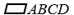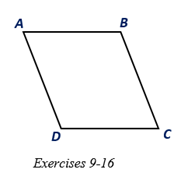Chapter 4.1, Problem 16EElementary Geometry For College St...

7th Edition
Alexander + 2 others
ISBN: 9781337614085

Solutions

Chapter
SectionElementary Geometry For College St...

7th Edition
Alexander + 2 others
ISBN: 9781337614085
Textbook Problem

Suppose that diagonals A C -   a n d   B D - ofare drawn and that A C > B D . Which angle ( ∠ A or ∠ B ) would have the greater measure?To determine

To describe:

The larger angle between A and B if AC>BD in.

Explanation

Given:

Diagonals AC- and BD- of are drawn and that AC>BD.

Corollary:

1. The opposite angles of a parallelogram are congruent.

2. Two consecutive angles of a parallelogram are supplementary.

3. The angle opposite to the larger side is larger than the angle opposite to the smaller side.

Calculation:

In the given parallelogram ABCD, given that AC>BD.

Join the diagonals AC- or BD- in

Still sussing out bartleby?

Check out a sample textbook solution.

See a sample solution

The Solution to Your Study Problems

Bartleby provides explanations to thousands of textbook problems written by our experts, many with advanced degrees!

Get Started

Calculate y'. 40. xey = y 1

Calculus: Early Transcendentals

Under what circumstances is a t statistic used instead of a z-score for a hypothesis test?

Essentials of Statistics for The Behavioral Sciences (MindTap Course List)

For f(x) = 5 + g(x), f(x) = _____. a) 5g(x) b) 5 + g(x) c) 0 g(x) d) g(x)

Study Guide for Stewart's Single Variable Calculus: Early Transcendentals, 8th